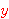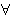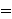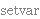New Foundations Explorer < Previous   Next > Nearby theorems Mirrors  >  Home  >  NFE Home  >  Th. List  >  ax-9 Unicode version

Axiom ax-9 1654
 Description: Axiom of Existence. One of the equality and substitution axioms of predicate calculus with equality. This axiom tells us is that at least one thing exists. In this form (not requiring thatandbe distinct) it was used in an axiom system of Tarski (see Axiom B7' in footnote 1 of [KalishMontague] p. 81.) It is equivalent to axiom scheme C10' in [Megill] p. 448 (p. 16 of the preprint); the equivalence is established by ax9o 1950 and ax9from9o 2148. A more convenient form of this axiom is a9e 1951, which has additional remarks. Raph Levien proved the independence of this axiom from the other logical axioms on 12-Apr-2005. See item 16 at http://us.metamath.org/award2003.html 1951. ax-9 1654 can be proved from the weaker version ax9v 1655 requiring that the variables be distinct; see theorem ax9 1949. ax-9 1654 can also be proved from the Axiom of Separation (in the form that we use that axiom, where free variables are not universally quantified). See theorem ax9vsep in set.mm. Except by ax9v 1655, this axiom should not be referenced directly. Instead, use theorem ax9 1949. (Contributed by NM, 5-Aug-1993.) (New usage is discouraged.)
Assertion
Ref Expression
ax-9Detailed syntax breakdown of Axiom ax-9
StepHypRef Expression
1 vx . . . . 52 vy . . . . 531, 2weq 1643 . . . 443wn 3 . . 354, 1wal 1540 . 265wn 3 1Colors of variables: wff setvar class This axiom is referenced by:  ax9v  1655
 Copyright terms: Public domain W3C validator